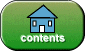created: Jan. 13, 2020

# Quiz on printf()

Instructions: For each question, choose the single best answer. Make your choice by clicking on its button.

1. Which of the following is the most general form of `printf()`

A.    ``` printf(locale, formatString, value1, value2, value3, ...) ```

B.    ``` printf(locale, formatString ) ```

C.    ``` printf( value1, value2, value3, ...) ```

D.    ``` printf( format1, format2, format3, ...) ```

2. What does the following print?

``` double value= -486.1234; System.out.printf("value=%8.2f%n", value);```

(The small dots ....in some answers show where spaces are printed.)

A.

`value=-486.1234`

B.

`value= -486.12`

C.

`value= -486.12n`

D.

`value=....-486.12`

3. What does the following print?

``` double value= 486.1999; System.out.printf("value=%10.1f%n", value); ```

A.

`value=......486.1`

B.

`value=....486.1999`

C.

`value=.....486.2`

D.

`value=486.12`

4. What does the following print?

``` int sum = 3487; System.out.printf("sum=%10d%n", sum); ```

A.

`sum=......3487`

B.

`sum= 3487`

C.

`sum=..........3487`

D.

`sum=3487.0`

5. What does the following print?

``` int sum = 3487; System.out.printf("sum=%010d%n", sum); ```

A.

`sum=0000003487`

B.

`sum=03487`

C.

`sum=00000000003487`

D.

`sum=03487`

6. What does the following print?

``` int sum = 3487174; System.out.printf("sum=%3d%n", sum); ```

A.

`sum=348`

B.

`sum=174`

C.

`sum=3487174`

D.    An Exception will halt the program.

7. What does the following print?

``` int sum = 10034; System.out.printf("sum=%8.2f%n", sum); ```

A.

`sum=10034.00`

B.

`sum=...10034`

C.

`sum=00010034`

D.    An Exception will halt the program.

8. What does the following print?

``` int balance=987654321; System.out.printf(Locale.US, "balance:%,12d%n", balance); ```

A.

`balance: 987654321`

B.

`balance: 987 654 321`

C.

`balance: 987,654,321`

D.

`balance:...987,654,321`

9. What does the following print?

``` String coins="dimes"; int number=19 ; double unitValue=0.10; System.out.printf( "You have %d %s worth %5.2f dollars%n", number, coins, number*unitValue ); ```

A.

`You have 19 dimes worth..1.90 dollars`

B.

`You have19dimes worth1.90dollars`

C.

`You have...19...dimes worth....1.90 dollars`

D.

`You have 19....dimes....worth  1.9 dollars`

10. What does the following print?

``` System.out.printf( "|%-10s%10s|%n", "Hello", "World" ); ```

A.

`|HelloWorld|`

B.

`|Hello.....World.....|`

C.

`|Hello..........World|`

D.

`| Hello World |`If you have returned here from another page, or have re-loaded this page, you will need to click again on each of your choices for the grading program to work correctly. You may want to press the SHIFT KEY while clicking to clear the old answers.